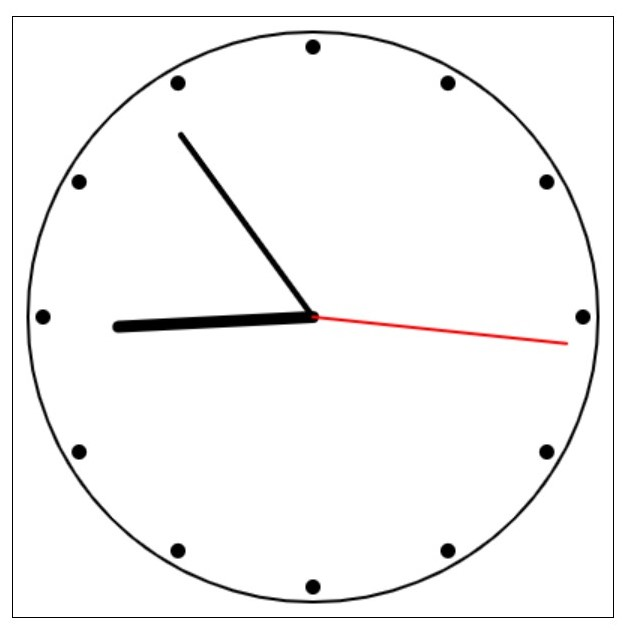# Programming Tutorials

## Sample Java Script that displays a movable clock

By: Rajan in Java Tutorials on 2006-12-11

This code creates a movable clock using HTML5 Canvas and JavaScript. The clock has hour, minute, and second hands that move to display the current time. The clock can also be moved by clicking and dragging on the canvas.

The HTML code creates a canvas element with an id of "canvas" and includes a JavaScript file named "movable-clock.js". The canvas is styled to be positioned in the center of the page with a black border.

To test it, create two files in the same folder. First create movable-clock.html with the below code.

```<!DOCTYPE html>
<html>
<title>Movable Clock</title>
<style>
#canvas {
position: absolute;
left: 50%;
top: 50%;
transform: translate(-50%, -50%);
border: 1px solid black;
}
</style>
<body>
<canvas id="canvas" width="400" height="400"></canvas>
<script src="movable-clock.js"></script>
</body>
</html>```

Then create another file movable-clock.js in the same folder and copy and paste the below code.

```// get the canvas element
var canvas = document.getElementById("canvas");

// get the initial position of the canvas
var initialX = 0;
var initialY = 0;

// add event listener for mouse down event
// set the initial position of the canvas
initialX = e.clientX;
initialY = e.clientY;

// add event listener for mouse move event
});

// add event listener for mouse up event
// remove event listener for mouse move event
document.removeEventListener("mousemove", movecanvas);
});

// function to move the canvas
function movecanvas(e) {
// calculate the new position of the canvas
var newX = initialX - e.clientX;
var newY = initialY - e.clientY;

// set the new position of the canvas
canvas.style.left = canvas.offsetLeft - newX + "px";
canvas.style.top = canvas.offsetTop - newY + "px";

// set the new initial position of the canvas
initialX = e.clientX;
initialY = e.clientY;
}

// get the canvas context
var canvas = document.getElementById("canvas");
var ctx = canvas.getContext("2d");

function drawcanvas() {
// clear canvas
ctx.clearRect(0, 0, canvas.width, canvas.height);

var centerX = canvas.width / 2;
var centerY = canvas.height / 2;
var radius = canvas.width / 2 - 10;

// draw canvas face
ctx.beginPath();
ctx.arc(centerX, centerY, radius, 0, 2 * Math.PI);
ctx.strokeStyle = "black";
ctx.lineWidth = 2;
ctx.stroke();

// draw marks
for (var i = 0; i < 12; i++) {
var angle = i * (Math.PI / 6);
var x = centerX + Math.sin(angle) * (radius - 10);
var y = centerY - Math.cos(angle) * (radius - 10);
ctx.beginPath();
ctx.arc(x, y, 5, 0, 2 * Math.PI);
ctx.fillStyle = "black";
ctx.fill();
}

// draw hands
var d = new Date();
var hour = d.getHours();
var minute = d.getMinutes();
var second = d.getSeconds();
var hourAngle = (hour % 12) * (Math.PI / 6) + (minute / 60) * (Math.PI / 6) + (second / 3600) * (Math.PI / 6);
var minuteAngle = minute * (Math.PI / 30) + (second / 1800) * (Math.PI / 30);
var secondAngle = second * (Math.PI / 30);

// draw hour hand
ctx.beginPath();
ctx.moveTo(centerX, centerY);
ctx.lineTo(centerX + Math.sin(hourAngle) * (radius - 60), centerY - Math.cos(hourAngle) * (radius - 60));
ctx.strokeStyle = "black";
ctx.lineWidth = 8;
ctx.lineCap = "round";
ctx.stroke();

// draw minute hand
ctx.beginPath();
ctx.moveTo(centerX, centerY);
ctx.lineTo(centerX + Math.sin(minuteAngle) * (radius - 40), centerY - Math.cos(minuteAngle) * (radius - 40));
ctx.strokeStyle = "black";
ctx.lineWidth = 4;
ctx.lineCap = "round";
ctx.stroke();

// draw second hand
ctx.beginPath();
ctx.moveTo(centerX, centerY);
ctx.lineTo(centerX + Math.sin(secondAngle) * (radius - 20), centerY - Math.cos(secondAngle) * (radius - 20));
ctx.strokeStyle = "red";
ctx.lineWidth = 2;
ctx.lineCap = "round";
ctx.stroke();

// request animation frame
requestAnimationFrame(drawcanvas);
}

// draw the canvas every second
setInterval(drawcanvas, 1000);```

Now open the movable-clock.html in your browser and you should see something like below.